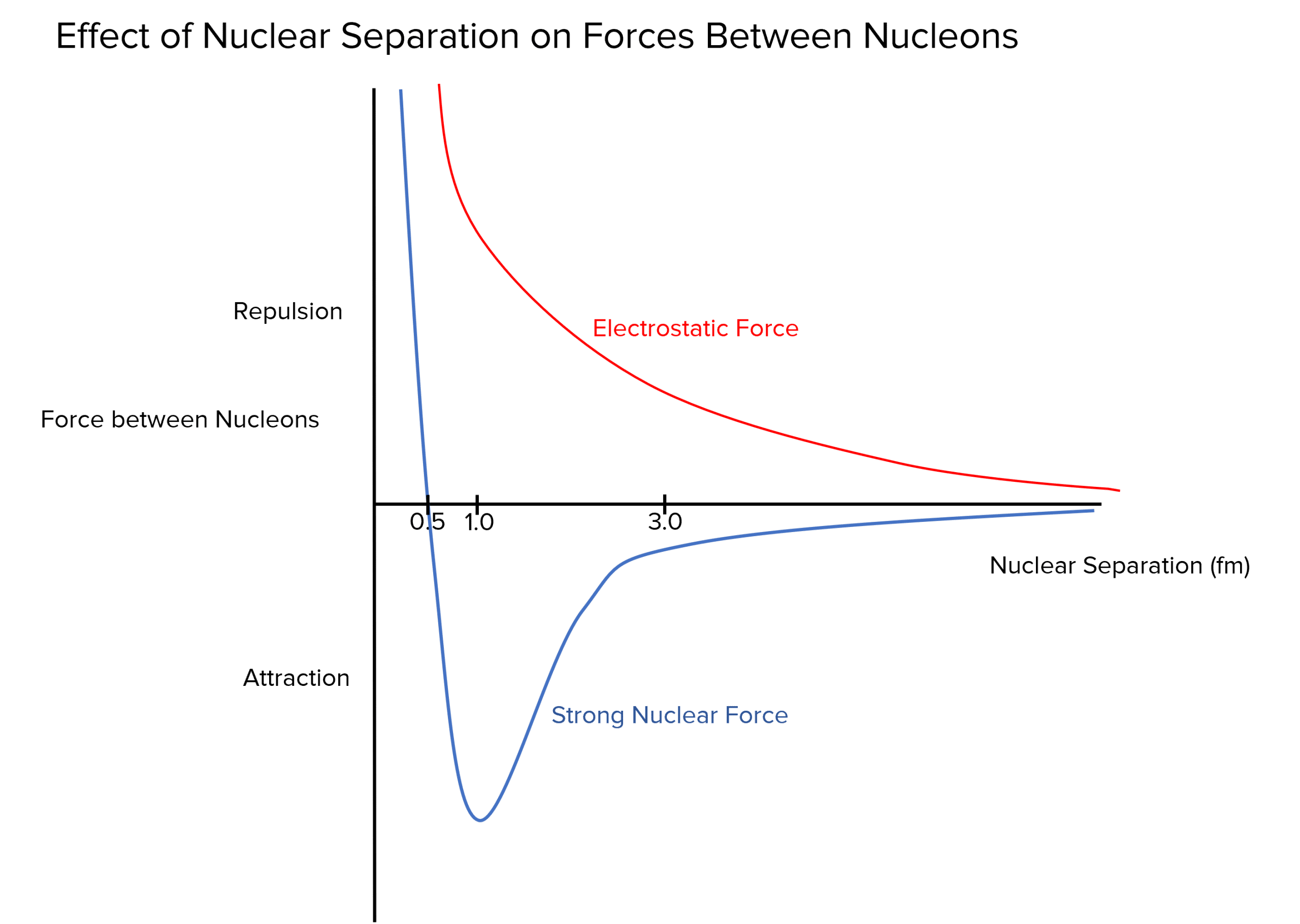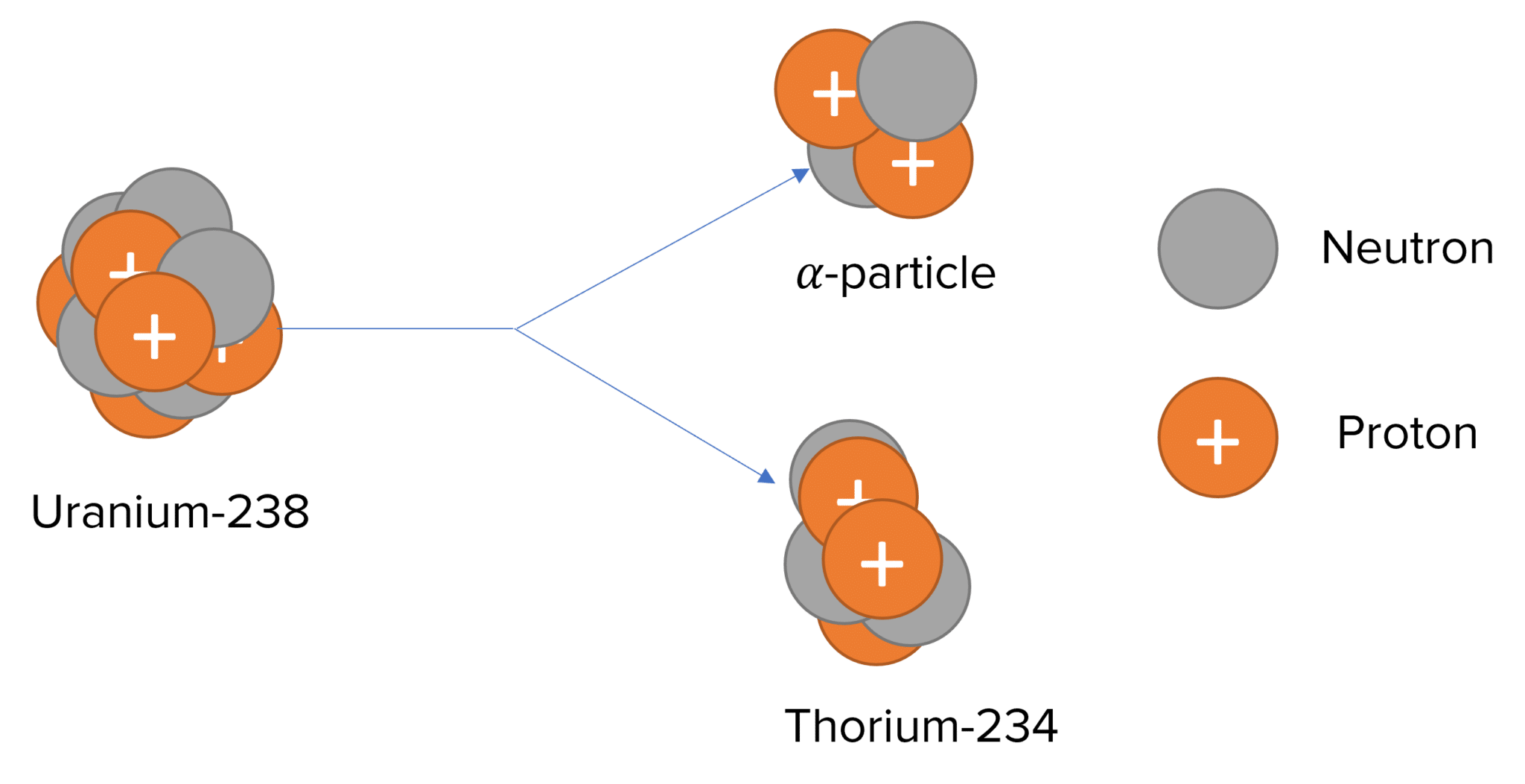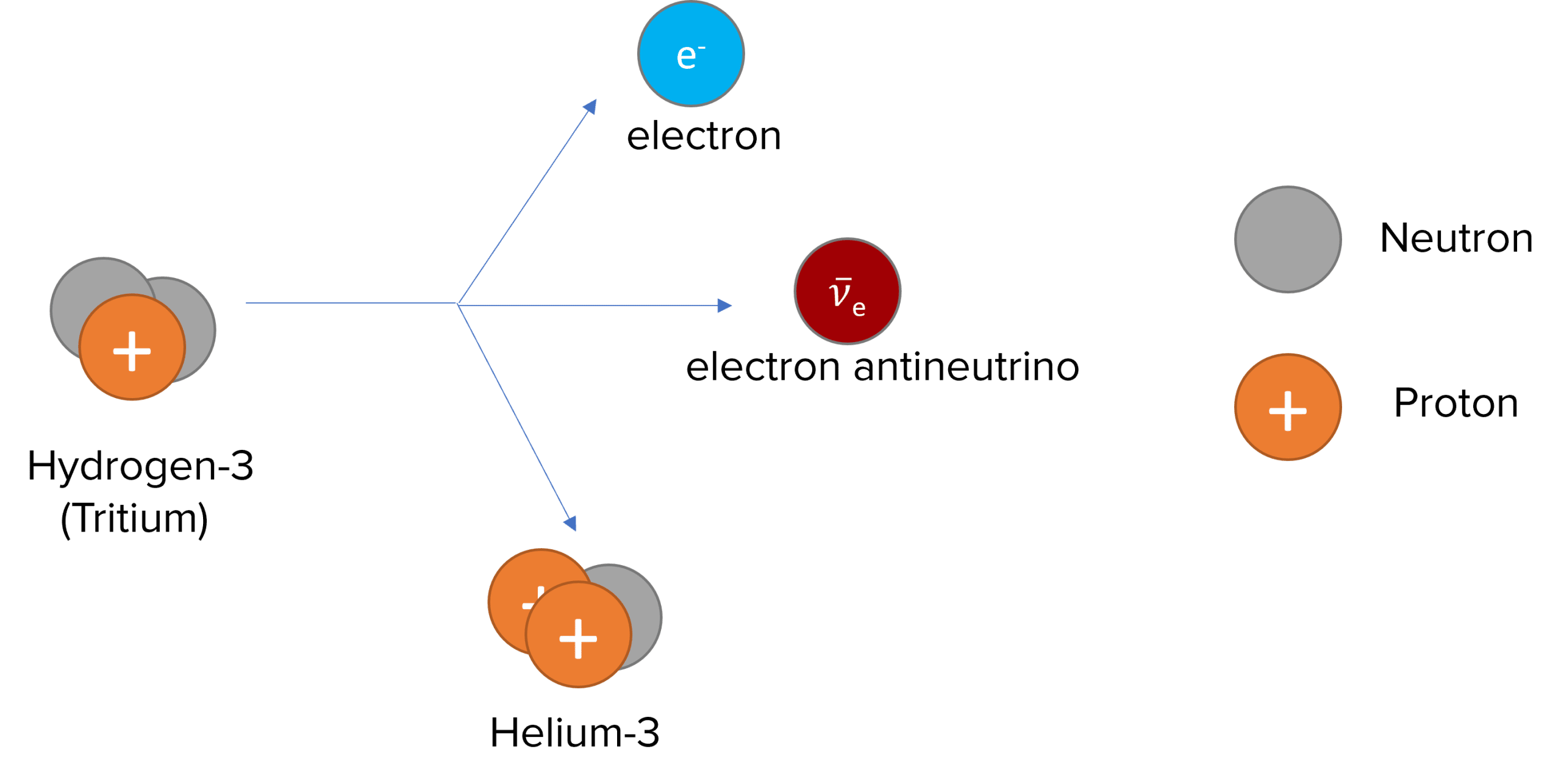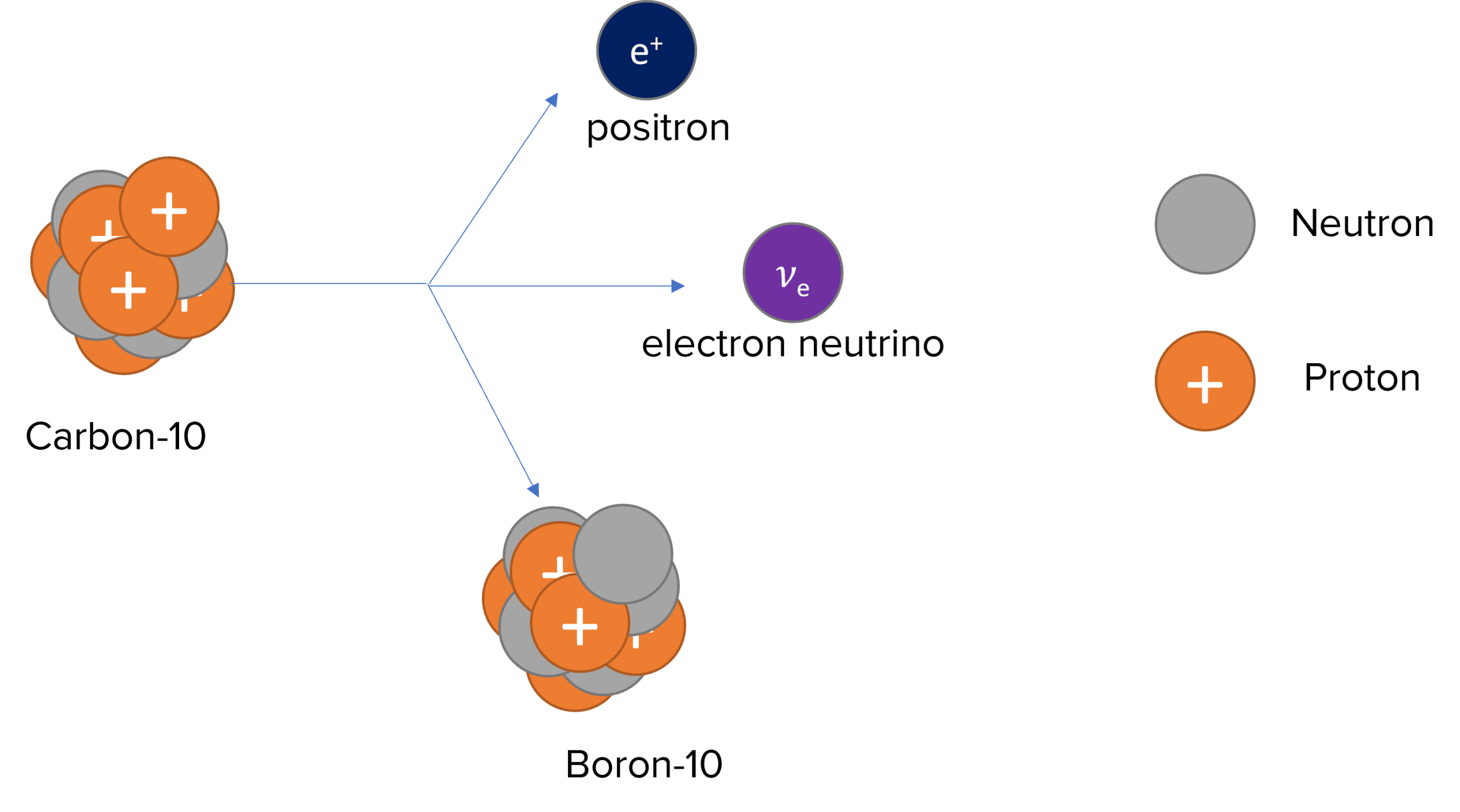# Stable and Unstable Nuclei

A LevelAS LevelAQA

## Stable and Unstable Nuclei

The strong nuclear force ensures the nucleons are bound together to create a stable nucleus. However, if the nucleus becomes unstable, then the nucleus composition can change through alpha or beta decay to become more stable.

## Strong Nuclear Force

Protons and neutrons (known as nucleons) are found inside the nucleus.

Protons are positively charged, therefore experience electrostatic repulsion. Neutrons are neutral, hence do not experience any electrostatic force.

Nucleons have masses therefore are gravitationally attracted to one another. However, the gravitational force between nucleons is much smaller than the electrostatic repulsion.

In order to keep the nucleus together, a third force is needed: the strong nuclear force.

The strong nuclear force holds quarks together and since both neutrons and protons contain quarks, the strong force works equally between all nucleons to keep the nucleus together.

The strong nuclear force varies depending on distance $\left(1 \: \text{fm} = 1×10^{-15} \: \text{m}\right)$:

• Less than $0.5 \: \text{fm}$: The strong nuclear force is repulsive to ensure the nucleus is not crushed.
• Between $0.5 \: \text{fm}$ and $3 \: \text{fm}$: The strong nuclear force is attractive and larger than the electrostatic repulsion.
• After $3 \: \text{fm}$: The strong nuclear force quickly falls to zero and has no effect.

The maximum value of attraction for the strong nuclear force occurs around $1 \: \text{fm}$, which is the average separation between nucleons in a nucleus (nuclear separation).

The strong nuclear force is also known as the strong interaction.

We can look at how the nuclear separation between nucleons effects the strong nuclear force between these nucleons.Note how when the nuclear separation is at around $1 \: \text{fm}$ in the graph, the strong nuclear force between the nucleons is at a maximum attraction.

A LevelAS LevelAQA

## Alpha Decay

For very large nuclei (e.g. Uranium, Radium), alpha decay is one of the most common forms of decay. This type of decay occurs because nuclei are too large for the strong nuclear force to hold them together.

Alpha decay involves the nucleus (the parent nucleus) releasing an alpha particle and decaying into a smaller nucleus, known as a daughter nucleus.

An alpha particle has 2 protons and 2 neutrons, the same as the nucleus of a Helium atom. The daughter nucleus has 2 less protons and 4 less nucleons compared to the parent nucleus. This results in a new element.

The general nuclear equation for alpha decay is:

$\textcolor{f21cc2}{ _Z^A X \rightarrow _{Z-2}^{A-4}Y + _2^4\alpha}$

As an example, Uranium-238 undergoes alpha decay to produce Thorium-234 and an alpha particle:

$^{238}_{92} U \rightarrow ^{234}_{90} Th + _2^4\alpha$A LevelAS LevelAQA

## Beta-Minus Decay

Beta-minus decay usually occurs when a nucleus is ‘neutron-rich’, meaning it has a large ratio of neutrons to protons.

It involves a neutron turning into a proton and an electron, and an anti-electron neutrino being released. The electron is the same as a Beta particle.

The proton number increases by one, but the nucleon number remains the same.

The general nuclear equation for beta-minus decay is:

$\textcolor{00bfa8}{ _Z^A X \rightarrow _{Z+1}^{A}Y + _{-1}^0\beta + \bar{v}}$

An example of beta-minus decay is Hydrogen-3 (Tritium) decaying to Helium-3 and releasing an electron and an electron antineutrino:

$_1^3 H \rightarrow \: _2^3 He +\: _{-1}^{0}\beta + \: _0^0 \bar{v_e}$A LevelAS LevelAQA

## Beta-Plus Decay

Beta-plus decay usually occurs when a nucleus is ‘proton-rich’, meaning it has a large ratio of protons to neutrons.

It involves a proton turning into a neutron and a positron (Beta-plus particle) and an electron neutrino being released.

The proton number decreases by one, but the nucleon number remains the same.

The general nuclear equation for beta-plus decay is:

$\textcolor{10a6f3}{ _Z^A X \rightarrow \: _{Z-1}^{A}Y + _{+1}^0\beta + v}$

An example of beta-plus decay is Carbon-10 decaying to Boron-10 and releasing a positron and an electron neutrino:

$^{10}_6 C \: \rightarrow ^{10}_5 B + _{+1}^0 \beta + v_e$A LevelAS LevelAQA

## Neutrinos

Neutrinos are sub-atomic particles that have no charge and negligible mass.

They are emitted from the nucleus during beta decay.

There are multiple types of neutrinos, however you only need to know two types: the electron neutrino $\left(v_e\right)$ and muon neutrino $\left(v_{\mu}\right)$.

Both types of neutrinos have an anti-particle version: electron antineutrino $\left(\bar{v}_e\right)$and muon antineutrino $\left(\bar{v}_{\mu}\right)$.

Neutrinos were initially hypothesised to ensure that energy was conserved during a beta decay. It was first thought that electrons were the only particle emitted during beta decay. However it was found that the total energy after the decay was less than the total energy before the decay. To solve this problem, Wolfgang Pauli suggested another particle also carried energy away, which had no charge (to ensure conservation of charge) and no/negligible mass (as it has not been detected). The neutrino was subsequently observed, affirming Pauli’s original hypothesis.

A LevelAS LevelAQA

## Stable and Unstable Nuclei Example Questions

A. Beta-minus decay

B. Beta-plus decay

C. Alpha decay

A nucleus contains protons and neutrons. The protons electrostatically repel each other and this force is larger than the gravitational attraction due to the mass of the nucleons.

Between $\boldsymbol{0.5} \: \textbf{fm}$ and $\boldsymbol{3} \: \textbf{fm}$, the strong nuclear force is an attractive force that holds the nucleons together.

Below $\boldsymbol{0.5} \: \textbf{fm}$, the strong nuclear force is repulsive to prevent the nucleons crushing, while above $3 \: \text{fm}$, the strong nuclear force goes to $0 \: \text{N}$.

Beta-plus decay

## You May Also Like...### MME Learning Portal

Online exams, practice questions and revision videos for every GCSE level 9-1 topic! No fees, no trial period, just totally free access to the UK’s best GCSE maths revision platform.

£0.00